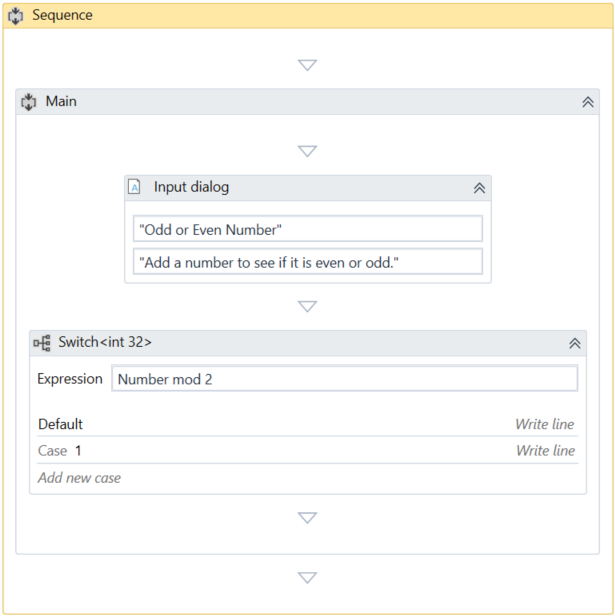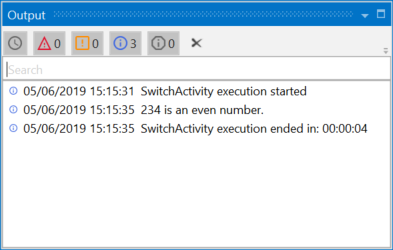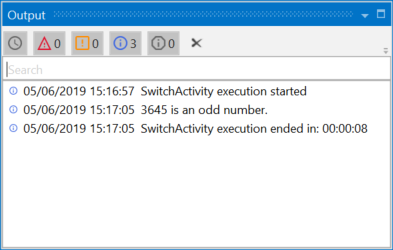Subscribe

# The Switch Activity

The Switch activity enables you to select one choice out of multiple, based on the value of a specified expression.

By default, the Switch activity uses the integer argument, but you can change it from the Properties panel, from the TypeArgument list.

The Switch activity can be useful to categorize data according to a custom number of cases. For example, you can use it to store data into multiple spreadsheets or sort through names of employees.

# Example of Using a Switch Activity

To exemplify how to use the Switch activity, we are going to create an automation that asks the user for a number, checks if is odd or even, and depending on that, a different message is written to the Output panel.

Since all odd numbers divided by two have a remainder equal to 1, this project needs only two cases (0 and 1) , yet keep in mind that this activity supports multiple cases.

1. Create a new sequence.
2. Create an integer variable, `Number`.
3. Add an Input Dialog activity to the Designer panel.
4. Add a Title and Label to prompt the user for a number.
5. In the Result field, add the `Number` variable.
6. Add a Switch activity, under the Input Dialog.
7. In the Expression field, type `Number` mod 2. This verifies if the user’s number is divisible by 2.
8. In the Default section, add a Write Line activity.
9. In the Text field, type `Number.ToString` + " is an even number.".
10. Click the Add new case line, and in the Case Value field, type 1.
11. Add a Write Line activity to this case.
12. In the Text activity, type `Number.ToString` + " is an odd number.".

The final project should look as in the following screenshot.1. Press F5. The automation is executed. Note that the Output panel displays the data correctly.Updated about a year ago